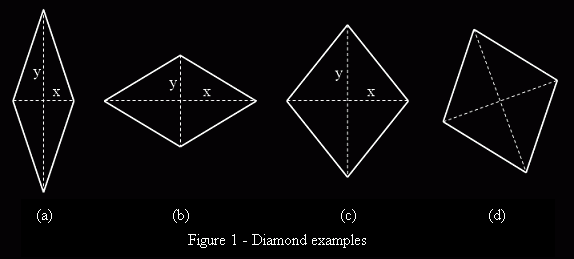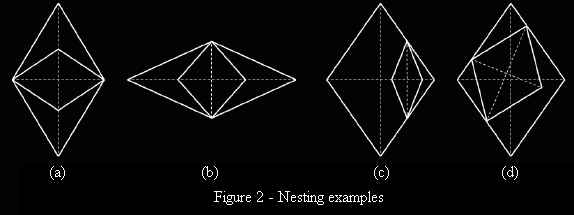### Problem Statement

A diamond is a quadrangle with 4 sides of equal length. In this problem, every diamond must be oriented so that one of its diagonals is strictly horizontal and the other is strictly vertical. For example, the diamonds shown in figures 1a, 1b and 1c below are oriented correctly, but figure 1d is not. The horizontal diagonal is called x and the vertical diagonal is called y. Please note that either x or y can be of length 0 (see example 4 for further clarification). There are two categories of diamonds: tall and wide. A diamond is called tall if y >= x, and a diamond is called wide if x >= y. The diamond in figure 1a is tall, and the diamond in figure 1b is wide. In figure 1c, x is equal to y, so you can choose to call the diamond tall or wide.You want to nest a set of diamonds one inside another. A diamond A is nested in diamond B if exactly two vertices of A are located strictly inside diamond B, and the other two vertices of A coincide with two different vertices of diamond B. Figures 1a and 1b show correctly nested diamonds, and figures 1c and 1d show incorrectly nested diamonds.For the purposes of this problem, a diamond A is considered nested in diamond B only if A is immediately nested in B. In other words, there must be no other diamonds between them. For example, if a diamond C is nested in diamond A, and diamond B is nested in diamond C, then B is not nested in A. The nesting of the set of diamonds must satisfy all of the following rules:

1. Each diamond must be used in the nesting exactly once.
2. Exactly one of the diamonds must be nested in none of the other diamonds. This diamond is called the outside diamond.
3. Each diamond, except the outside diamond, must be nested in exactly one other diamond.
4. Each tall diamond must be nested in a wide diamond.
5. Each wide diamond, except the outside diamond, must be nested in a tall diamond.
6. The outside diamond must be wide.

The height of the nesting is equal to the length of the vertical diagonal of the outside diamond. You are given a int[] sides, where each element is the side length of a single diamond in the set. Return the minimum possible height of their nesting. If it is impossible to create a valid nesting, return -1 instead.

### Definition

 Class: NestedDiamonds Method: minHeight Parameters: int[] Returns: double Method signature: double minHeight(int[] sides) (be sure your method is public)

### Notes

-A return value with either an absolute or relative error of less than 1.0E-9 is considered correct.

### Constraints

-sides will contain between 1 and 50 elements, inclusive.
-Each element of sides will be between 1 and 1,000,000, inclusive.

### Examples

0)

 `{4, 2}`
`Returns: 2.8284271247461903`
1)

 ```{10, 5, 2} ```
`Returns: 9.16515138991168`
2)

 `{1,2,5,3}`
`Returns: 4.69041575982343`
 The side lengths of the diamonds in their nested order, starting from the outside diamond, are 5, 3, 2, 1.
3)

 `{1, 1}`
`Returns: -1.0`
 A diamond cannot be nested in another diamond with the same side length.
4)

 `{1,4,3}`
`Returns: 5.656854249492381`
 The height of the innermost diamond is 0.

#### Problem url:

http://www.topcoder.com/stat?c=problem_statement&pm=7817

#### Problem stats url:

http://www.topcoder.com/tc?module=ProblemDetail&rd=10802&pm=7817

gevak

#### Testers:

PabloGilberto , Yarin , Olexiy , ivan_metelsky

Math, Search